### Revising IGCSE Maths? Here are 4 types of Triangle Questions you need to master

No matter how hard you TRI, when studying IGCSE (or GCSE) Maths it can be difficult to stay on top of all the different versions of triangle questions.

From Pythagoras’ Theorem to the Cosine Rule, students often complain that half the exam paper is made up of tricky triple-pointed problems!

But help is at hand. The Save My Exams Maths team have scoured our IGCSE Revision Notes and Topic Questions, and they’ve pinpointed four different examples of triangle problems you need to get to grips with.

Better still, they’ve included the rules and tricks you need to know to solve each problem, as well as examples of how to put the rules into practice when solving exam questions.

If you’re looking up-level your Maths skills fast, you’re in the right place.#### Similar Triangles

Two triangles are mathematically ‘similar’ if they both have their equivalent angles are equal, and their equivalent lengths in the same ratio. This means that one triangle will be an enlargement of the other.

Questions on similar triangles will usually require you to calculate the scale factor of the enlargement.

You can do this by identifying equivalent known side lengths, and dividing the second length ÷ first length (Check that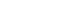if getting bigger andif getting smaller).

When you’ve calculated the scale factor, use it to work out any missing side lengths.

#### Example#### Solution

Step one: Identify that triangle ADC is and enlargement of triangle AEB

Step two: Identify two equivalent sides (CD and BE)

Step three: Calculate the scale factor (1.5 divided by 6  = 0.25)

Step four: Multiply AD by the same SF to calculate AE (5 x 0.25 = 1.25)

Step five: Subtract AE from AD to find ED (5 – 1.25 = 3.75)

Practice with our Similar Shapes Topic Questions

#### Pythagoras’ Theorem

Pythagoras’ Theorem tells us that, where a, b, and c are the lengths of the three sides of a right-angled triangle, and c is the side opposite the right angle (the hypotenuse),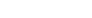As long as you know the lengths of two sides of the triangle, you can use this rule to work out the missing side length.

#### ExampleThis question requires you to use Pythagoras’ Theorem twice:

Step one: Calculate BD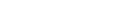so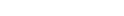Step two: Use BD and DC to calculate BC (x)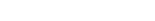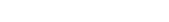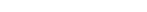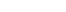Practice here

#### SOHCAHTOA

‘SOHCAHTOA’ rules are useful for calculating angles or lengths in a right-angled triangle, as long as you know two side lengths (for calculating angles) or one side and one angle (for calculating side lengths).

First, label the triangle: the angle you know (or want to know) is ‘θ’ , ‘O’ is the side opposite this angle, ‘H’ is the side opposite the right angle, and ‘A’ is the third side (adjacent to θ).Next, choose SOH, CAH, or TOA.

The letters of the sides you’re interested in (i.e. know or want to know) tell you which to choose:

• To find a side: put and the side you know into the equation, and solve to find the side you don’t know• To find an angle: use the inverse trig functions on your calculator:Ready to try the formulae out? Find practice questions with full answer explanations here

#### Sine Rule and Cosine Rule Questions

Pythagoras and SOHCAHTOA only help up to find missing side lengths and angles in right-angled triangles.

However, the Sine Rule and Cosine Rule help us solve questions involving ANY type of triangle

To use them correctly, label your triangle like in the below diagram (be careful not to confuse your upper and lowercase letters).The Sine Rule states that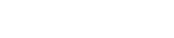andThe Cosine Rule (or ‘Cos Rule’) states that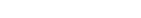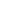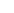andExampleStep one: Use the Sine Rule to calculate BD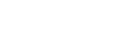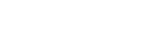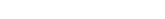Step two: Use the ANS value for BD (which we’ll call ‘c’) and the AB length (‘b’) to calculate AD (‘a’) with the Cosine Rule.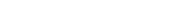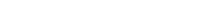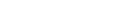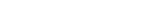Raring to go? Find more practice trigonometry questions and watch video solutions here

You’ve done a great job to make it to the end of this revision blog, but don’t stop now!

If you want to tick off some more topics from your revision list, there’s no time like the present.

Start by exploring the rest of our teacher-written Revision Notes for CIE and Edexcel IGCSE Maths.